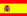You are here : > Home> Utilities > Mathematics & Statistics > Determinant - 3 by 3 matrix >

### Determinant - 3 by 3 matrix

Determinant is a number associated with a type of matrix known as square, where the number of rows and columns is equal.
A matrix is an ordered set of elements arranged in rows and columns, where "m" and "n" represent respectively rows and columns.
The matrices and determinants are found not only in mathematics, but also practical applications in engineering, computer science, finance etc .
To calculate the determinant of a 3 by 3 matrix or square matrix order 3, enter data as requested. Use the point as a decimal separator. Ex 2,533.34; enter 2533.34; The results are automatically displayed after you click on "Calculate"

 Determinant - 3 by 3 matrix Determinant: